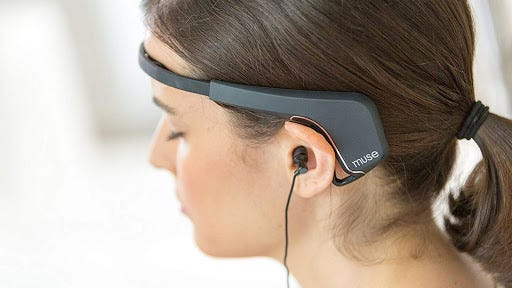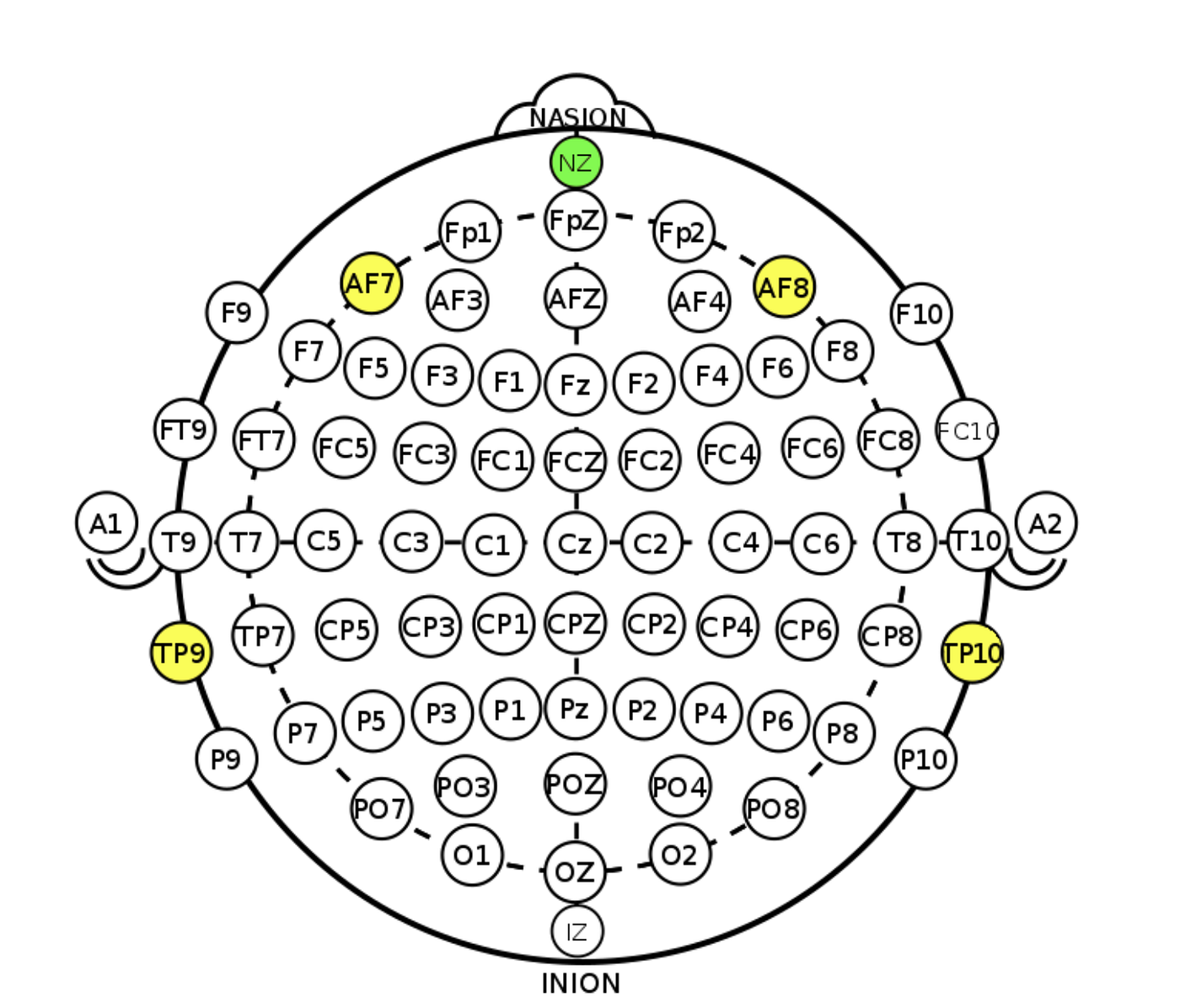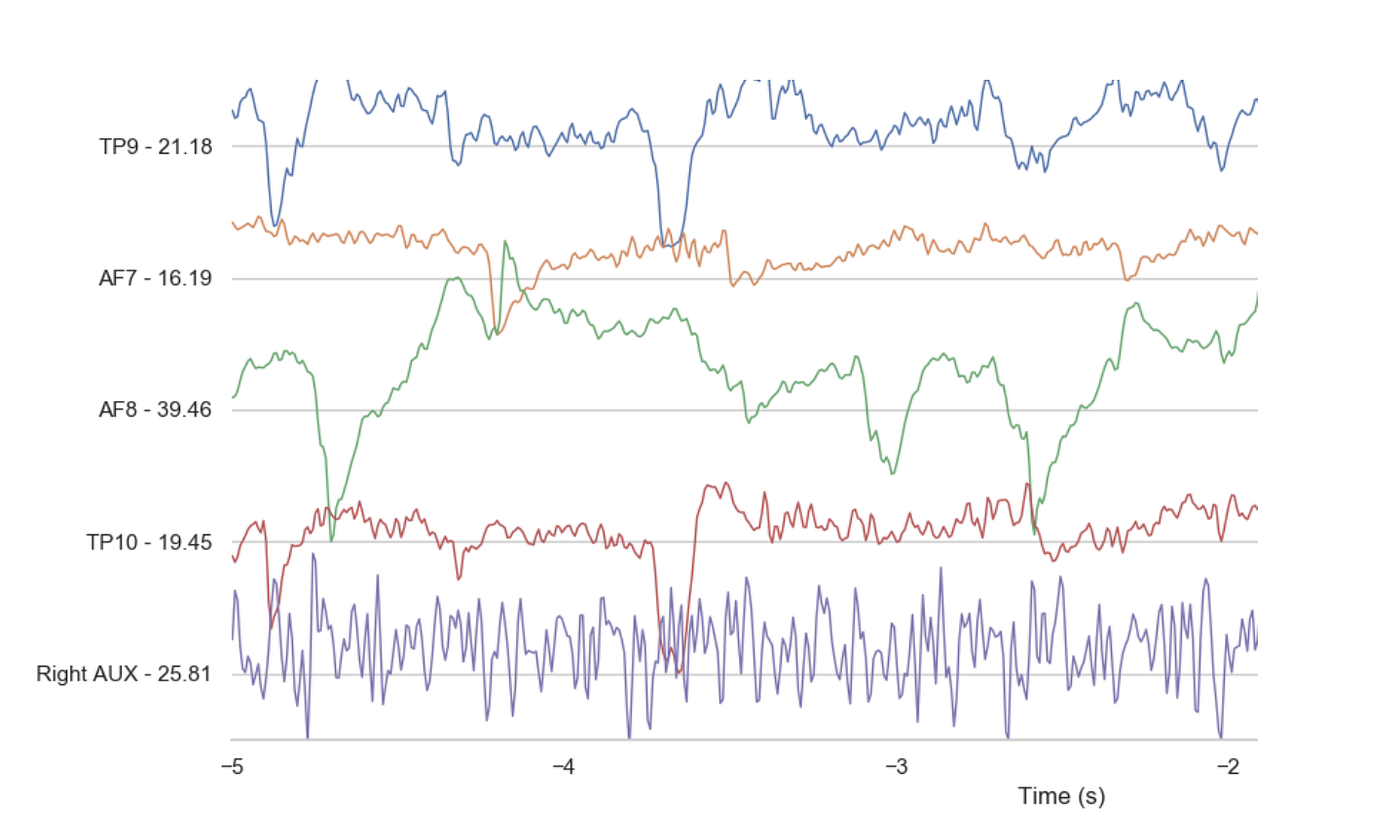# Mental Emotional Sentiment Classification Using Machine Learning and an Electroencephalogram (EEG)

A couple of days ago, I was trying to teach myself how to use AI to understand and classify brainwaves when I came across this very interesting study by Jordan J. Bird.

I have attached the official study, and an excellent youtube video he created at the end, as additional resources at the end of this article.

You can also find the resources linked here:
Study: http://jordanjamesbird.com/publications/A-Study-on-Mental-State-Classification-using-EEG-based-Brain-Machine-Interface.pdf

But for now, this article is to give you an understanding of the nitty-gritty behind the study and how you can replicate it for yourself.

If you don’t know what an EEG is:

Electroencephalogram (EEG): Is a non-invasive headset that reads your brain waves using electrodes (sensory nodes) at various points around your head.

Essentially, an EEG picks up on the electrical signals your brains give off as a result of conscious behavior and reports them.An example of an EEG by Muse (also happens to be the same one as used in the study). Credit to Muse.Credit to Muse.

If you’re more curious about EEGs and the specifics behind them, you can check out my article here.

The entire article can be broken down into the following sections.

1. An outline
2. Data Acquisition
3. Statistical Extraction
4. Feature Selection
5. Model + Results
6. Contact
7. Resources Used

# 1. An outline

The goal of this study is to be able to classify your brain state into happy, neutral, or sad states using 5 EEG signals (alpha, beta, theta, delta, gamma) as starting features in a machine learning algorithm. The study tested various combinations of feature selection algorithms and classification models to compare performance in terms of accuracy and the number of features needed. Through the use of the InfoGain selection algorithm and Random Forests model, they were able to get a result of nearly 98%. Is this article, however, I will be using Gabriel Atkin’s code you can find here because it is published alongside a tutorial that makes it easier to follow.

# 2. Data Acquisition

The MUSE headband was strapped with 4 electrodes in the following locations (Fig.1) TP9, AF7, AF8, and TP10, and recorded subjects while exposed to various stimuli.Fig. 1. The electrodes follow the following configuration. The green point is used as a reference point for calibration.

The stimuli, in this experiment, was the viewing of variously themed clips of movies. The valance correlates to the emotions of that scene. Neg is Negative and Pos is Positive.

For example, the clip from Marley and Me was a death scene while the clip from Funny Dogs evoked a physical smile from patients.

In addition to the stimulus listed below, data was collected without a stimulus to be used as the “neutral” dataset.

Blinking was not encouraged nor discouraged but physical movements such as moving one's arm were discouraged. Measures were taken to ensure that interference between physical facial movements and the EEG recording was minimal.

Subjects were not exposed to any stimuli to gain data for a neutral state first. The stimulus was activated a couple of seconds before the EEG was told to pick up the electrical waves in order to make sure that transitions did not count as labeled data.

The data was observed to be streaming at a variable frequency within the range of 150–270 Hz and all signals were recorded along with a UNIX timestamp, which was later used for downsampling to produce a uniform stream frequency. The end goal was to get something like this:Right Aux can be disregarded as unimportant.

The sampling rate was decimated to 200 Hz based on fast Fourier transformations along a given axis. Because the Fourier method is used, the signal is assumed to be periodic.

# 3. Statistical (Feature) Extraction

One of the hardest parts of feature extraction is interpreting the complexity of the brain signals. The signals are non-linear, non-stationary, and virtually random in small increments.

The signals are only considered stationary within short intervals, which is why the study opted to apply a short-time windowing technique to match this characteristic.

This subsection describes the set of features considered in this work to adequately discriminate different classes of mental states. These features rely on statistical techniques, time-frequency based on fast Fourier transform (FFT), Shannon entropy, max-min features in temporal sequences, log-covariance, and others. All features proposed to classify the mental states are computed in terms of the temporal distribution of the signal in a given time window. This sliding window is defined as a period of 1 second and is computed every 0.5 seconds. All of the following statistical extractions are applied to each of the 5 brainwaves which amounted to a total of 2147 features.

The following are the statistical extractions with the relevant code sourced from here:

Note: As we go through the process behind assembling the matrix, you will see large blocks of code. I highly encourage you to, at least, read the comments within the blocks to get a better idea of what the functions are doing.

First, we start with the necessary stuff:

Then,
Given a set of data values (being the stream of electrical activity from the headset): {x1, x2, …xN}

We slice the signal into 1s frames.

Then, we compute the following for each individual frame.

The mean value:

Mathematically represented as:

In code:

Then, we compute the mean for the first and second half of each frame and calculate the backward difference like so:

Then, we take things one step further, relative to individual quadrants of the single second frame:

Then, we move on to doing something similar with The Standard Deviation:

Then again, computing the backward difference of the 0.5-second frames within a single frame.

Now, we calculate The Statistical moments of 3rd and 4th order (another way of saying calculating skewness and kurtosis):

y = {skewness, k = 3; kurtosis, k = 4}.

Next, for every single frame (one second), the maximum and minimum values are computed. The code related to the min and max values only shows the code for calculating maximum values but there is additional code within the file that calculates for the minimum values. I chose not to include the code for minimum values because it is very similar.

along with the backward difference of the two half frames within the single frame

Then the paired differences of the max values for each quarter

Given the calculated features, we can assemble a square matrix to computer log-covariance as:

To understand log covariance, you first need to understand what a Covariance Matrix is. A covariance matrix computers the correlation between each of the features in the matrix with the other features in the matrix. The goal is to narrow down the number of features to avoid. In this case, we assemble a 12x12 matrix which means we are looking at 144x144 correlations. From here, it selects which features have the highest or lowest variance depending on the algorithm that will be run. Log Covariance is just a way of making this process less computationally intense for the computer.

In code, we first assemble a covariance matrix:

This allows us to calculate the Eigenvalues of the covariance matrix like so:

And then we can compute the log-covariance:

At this point, we’ve extracted most of the features to give a good understanding of the signal. There are a couple of features to extract before we are ready to assemble and finalize the matrix.

That includes running the signals through an algorithm called the Fast Fourier Transformation (FFT). FFT transforms a temporal signal to its frequency domain. It acts as a way to split up the signal into its constituent frequency bands and helps isolate the ones giving the most information.

We then bring all of the defined functions (to generate features) into one big vector.

Then we finish by creating one final function that reads in the data from the original CSV and pushes it through the above function calc_feature_vector.
All the functions above were definitions. generate_feature_vectors_from_samples is the function that connects the definitions to the data they will use to create data points to be used in the model.

and that’s it! We’ve now finalized all of the extracted features into one function. At this point, we can go from creating new features to assembling a finalized matrix for use in a model.

For this process, we create a new file of code. You can find it here.

# 4. Feature Arrangement

We start with the imports which include the final function from the previous file.

Then, we use the imported function to read the CSV file in and process it through the functions to assemble the training matrix and save it to a file:

For the sake of brevity, you don’t need to run the above code. You can find the finalized assembled matrix here. This matrix is a very large file with over 2500 dimensions so I would not recommend trying to create or open it on your personal computer. The file is called emotions.csv.

From here, however, we can plug this matrix into a Random Forest Classifier.

Note: The official study rules Random Forests as the best method of classification, which is why I’m writing about it. Bear in mind that’s doesn’t rule out other forms of classification. One form of classification I find particularly interesting is to project the EEG waves as images and use a Convolutional Neural Net to act as the model. This is mentioned in the Related Work + Next Steps portion of the study.

# 5. The Model + Results

You can find the source of the code I used here, but for the purpose of the article, I will be using my own version which you can find in a Google colab document here.

Most of the imports are fairly common.

The one that I was a bit unfamiliar with is the Random Forest Classifier. You can find the official documentation for the RandomForestClassifier here. Essentially, it automates the process of building the various trees.

Additionally, the model uses cross-validation to find the best combination of data to train the model. You can find the relevant documentation for that here and a good youtube explanation of what cross-validation is here.

The next step is to import the data and read it into the workspace.

Part of this involves printing the data head (first 5 datapoints) to check the format and take a look at what the data actually looks like.

We then return the dimensions of the matrix to get a full idea of how big the dataset is. In this case, it has 2132 datapoints with 2549 values associated with them.

We then take a look at the tendencies of the data.

The describe() function is used to generate descriptive statistics that summarize the central tendency, dispersion, and shape of a dataset’s distribution (excluding NaN values).

Now, before we run the model through the classifier, we need to make sure we have an equal distribution of data across Positive, Neutral, and Negative (classification) values, so the training process isn’t weighted to favor one output more than another.

We can check that the data is relatively even by building a plot like so:

You can see that the bars are relatively even, so we don’t need to alter the amount of data to each target variable to prevent any bias.

Now that we’ve troubleshooter any major flaws, we can go ahead and plug the data into a Random Forests Algorithm.

One of the advantages of Random Forests is it automatically reduces the feature set.

First, we rename the automated RandomForestClassifier Pipeline to pl_random_forest.

We then use the cross-validation function with a couple of directing inputs. If you’re unsure about any of the concepts I highly recommend looking at this youtube video and this documentation.

• pl_random_forest acts as the object used to fit the data when calculating scores.
• Label_df is the final vector of the data with the actual classification as positive, negative, or neutral.
• cv is the number of “folds” the data is divided into. In this case, cv is declaring that the data will go through 10-fold cross-validation to get the best combination of data + trees for the algorithm.
• accuracy is used as the function to evaluate the score of each setup undergoing cross-validation.

Then we print out the accuracy as the mean average of the scores calculated from cross-validation.

In this particular instance, we get a 98.5% accuracy, which exceeds the results from the actual study performed 🤯.

And that’s it! You can now replicate this project for yourself and classify your own mental-emotional sentiment with a little bit of code and an EEG. Given a stream of EEG waves, your model can now tell the difference between Positive, Neutral, and Negative emotions.

# Contact

Hey everyone, Thanks for reading this far! I hope you got something out of the article.
My name is Satvik. I’m a 15-year-old interested in Machine Learning, Brain-Computer-Interfaces, and philosophy. Feel free to contact me on any of the platforms below. I hope you enjoyed the article!
Email: satvikagnihotri12@gmail.com
Find my LinkedIn here.
You can also signup for my personal monthly newsletter here.
Let’s Chat! Book a call here.

--

--